Need help with tensorspace?
Click the “chat” button below for chat support from the developer who created it, or find similar developers for support.tensorspace-team4.5K Stars398 ForksApache License 2.0687 Commits23 Opened issues#### Description

Neural network 3D visualization framework, build interactive and intuitive model in browsers, support pre-trained deep learning models from TensorFlow, Keras, TensorFlow.js!
?

#### Need anything else?#### Contributors list# TensorSpace.js

Present Tensor in Space

English | 中文

TensorSpace is a neural network 3D visualization framework built using TensorFlow.js, Three.js and Tween.js. TensorSpace provides Keras-like APIs to build deep learning layers, load pre-trained models, and generate a 3D visualization in the browser. From TensorSpace, it is intuitive to learn what the model structure is, how the model is trained and how the model predicts the results based on the intermediate information. After preprocessing the model, TensorSpace supports to visualize pre-trained model from TensorFlow, Keras and TensorFlow.js.Fig. 1 - Interactive LeNet created by TensorSpace

## Motivation

TensorSpace is a neural network 3D visualization framework designed for not only showing the basic model structure, but also presenting the processes of internal feature abstractions, intermediate data manipulations and final inference generations.

By applying TensorSpace API, it is more intuitive to visualize and understand any pre-trained models built by TensorFlow, Keras, TensorFlow.js, etc. TensorSpace introduces a way for front end developers to be involved in the deep learning ecosystem. As an open source library, TensorSpace team welcomes any further development on visualization applications.

• Interactive -- Use Layer API to build interactive model in browsers.
• Intuitive -- Visualize the information from intermediate inferences.
• Integrative -- Support pre-trained models from TensorFlow, Keras, TensorFlow.js.

## Getting Started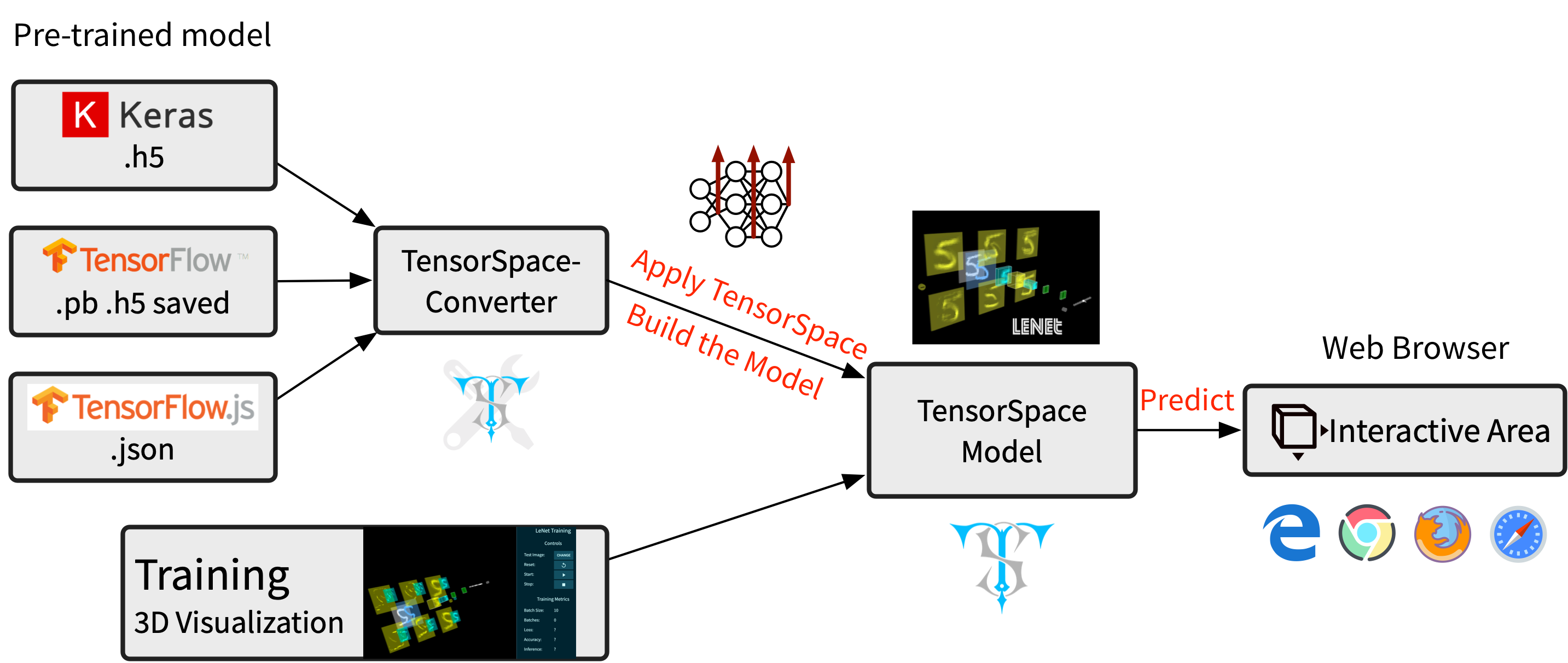Fig. 2 - TensorSpace Workflow

### 1. Install TensorSpace

#### Install in the Basic Case

Download dependencies build files TensorFlow.js (tf.min.js), Three.js (three.min.js), Tween.js (tween.min.js), TrackballControls (TrackballControls.js).

`tensorspace.min.js`
from Github, NPM, TensorSpace official website or CDN:

• Step 3: Include Build Files

Include all build files in web page.

```

```

#### Install in the Progressive Framework

• Step 1: Install TensorSpace

• Option 1: NPM
```  npm install tensorspace
```
• Option 2: Yarn
```  yarn add tensorspace
```
• Step 2: Use TensorSpace
```import * as TSP from 'tensorspace';
```

### 2. Preprocess the Pre-trained Model

Before applying TensorSpace to visualize the pre-trained model, there is an important pipeline - TensorSpace model preprocessing ( Checkout this article for more information about TensorSpace preprocessing ). We can use TensorSpace Converter to quickly complete the TensorSpace Preprocessing.

For example, if we have a tf.keras model in hand, we can use the following TensorSpace-Converter conversion script to convert a tf.keras model to the TensorSpace compatible format:

```shell
\$ tensorspacejs_converter \
--input_model_from="tensorflow" \
--input_model_format="tf_keras" \
./PATH/TO/MODEL/tf_keras_model.h5 \
./PATH/TO/SAVE/DIR
```

Note:

• Make sure to install
`tensorspacejs`
pip package, and setup a TensorSpace-Converter runtime environment before using TensorSpace-Converter to preprocess the pre-trained model.
• Based on different training libraries, we provide different preprocessing tutorials: TensorFlow Tutorial, Keras Tutorial, TensorFlow.js Tutorial.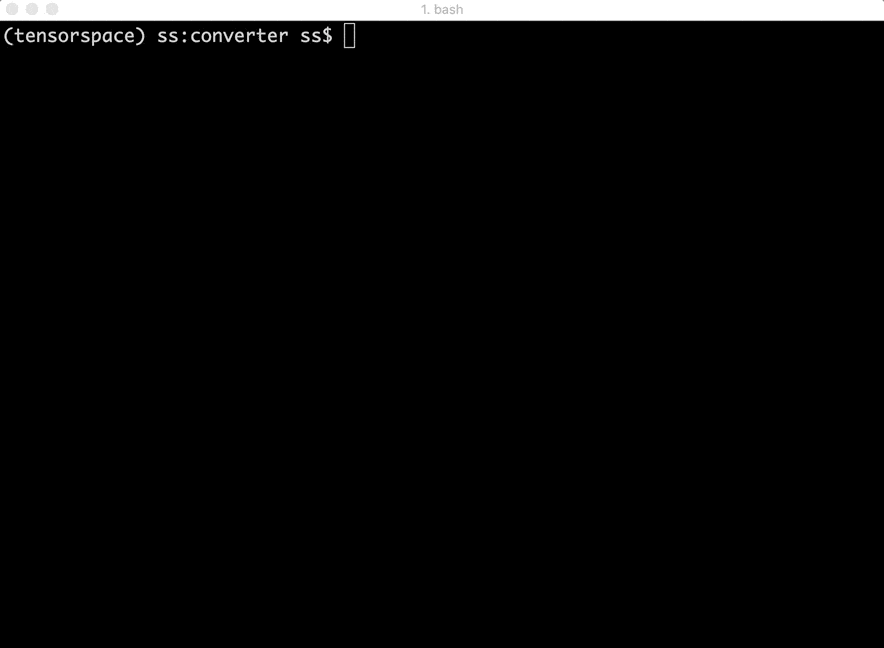Fig. 3 - TensorSpace-Converter Usage

### 3. Using TensorSpace to Visualize the Model

If TensorSpace is installed successfully and the pre-trained deep learning model is preprocessed, let's create an interactive 3D TensorSpace model.

For convenience, we will use the the resources from this repository's HelloWorld directory, which includes preprocessed TensorSpace compatible LeNet model and sample input data ("5") as an example to illustrate this step. All source code can be found in helloworld.html.

First, we need to new a TensorSpace model instance:

```JavaScript
let container = document.getElementById( "container" );
let model = new TSP.models.Sequential( container );
```

Next, based on the LeNet structure: Input + Padding2D + 2 X (Conv2D & Maxpooling) + 3 X (Dense), build the Topology of the TensorSpace model:

```JavaScript
outputs: ["0", "1", "2", "3", "4", "5", "6", "7", "8", "9"]
}) );
```

Last, we should load our preprocessed TensorSpace compatible model and use

`init()`
method to create the TensorSpace model:
```JavaScript
type: "tensorflow",
url: './PATH/TO/MODEL/model.json'
});
model.init(function(){
console.log("Hello World from TensorSpace!");
});
```

We can get the following Fig. 3 model in the browser if everything looks good.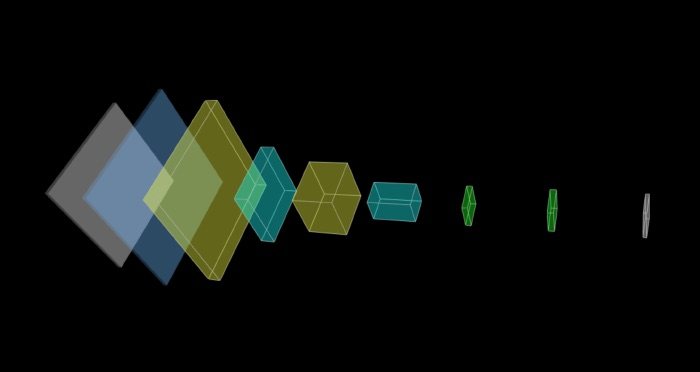Fig. 4 - LeNet model without any input data

We provide a extracted file which is a handwritten "5" as the input of our model: (online demo)

```model.init(function() {
model.predict( image_5 );
});

```

We put the

`predict( image_5 )`
method in the callback function of
`init()`
to ensure the prediction is after the initialization complete.

Click the CodePen logo to try it in CodePen: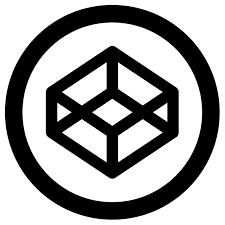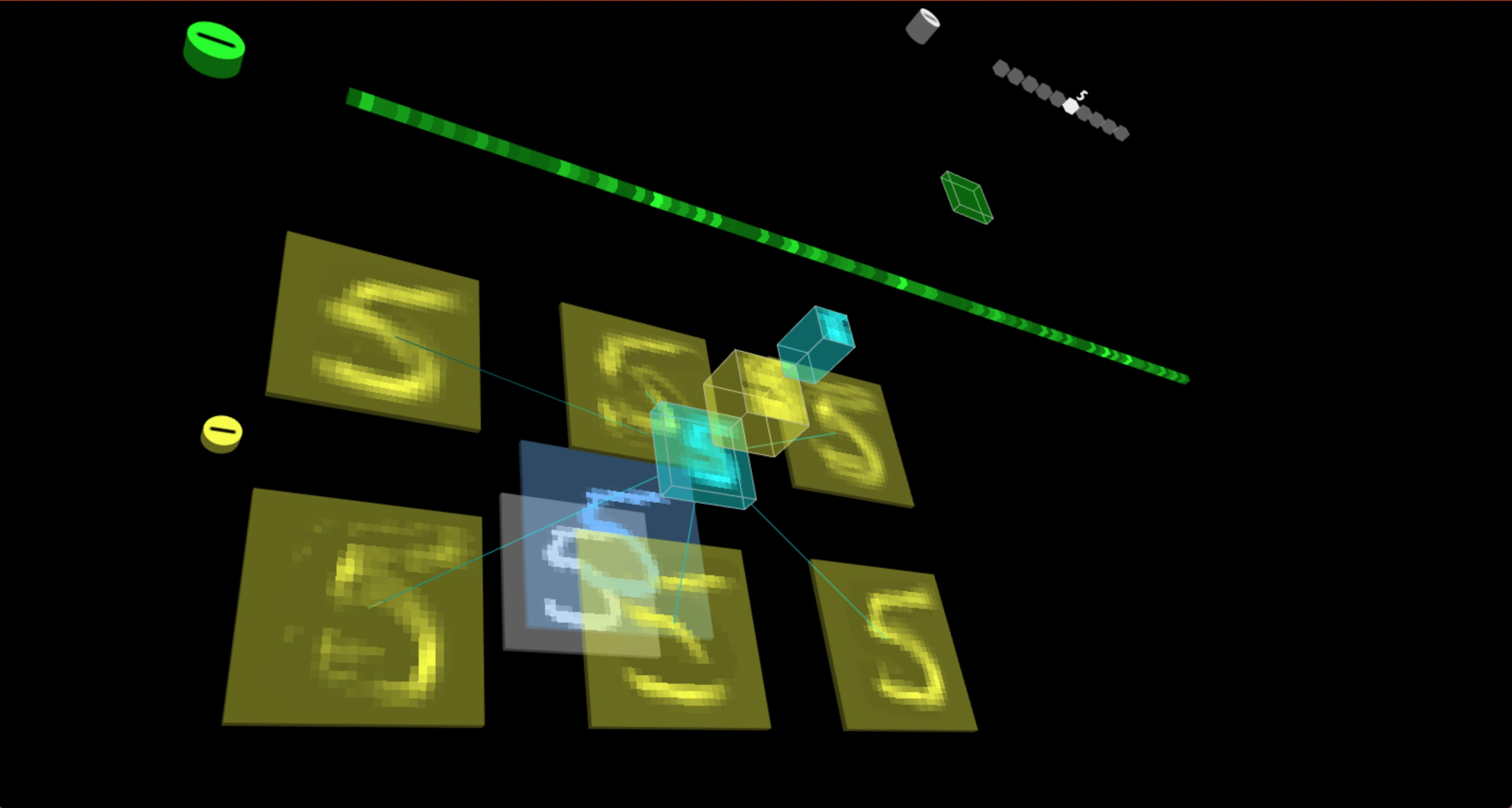Fig. 5 - LeNet model with input data "5"

## Example

• LeNet [ TensorFlow.js model ]

➡ Live DemoFig. 6 - Interactive LeNet created by TensorSpace

• AlexNet [ TensorFlow model ]

➡ Live Demo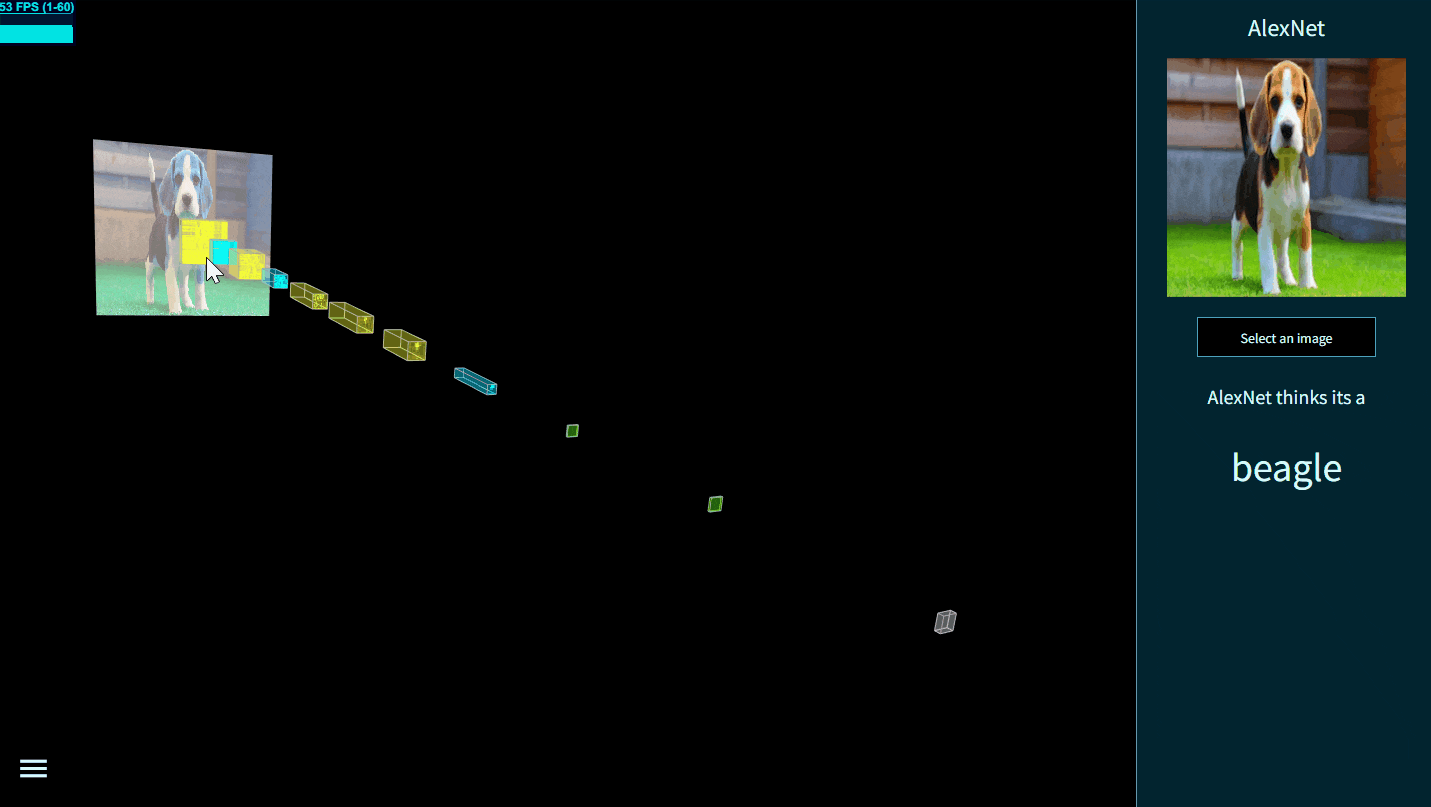Fig. 7 - Interactive AlexNet created by TensorSpace

• Yolov2-tiny [ TensorFlow model ]

➡ Live Demo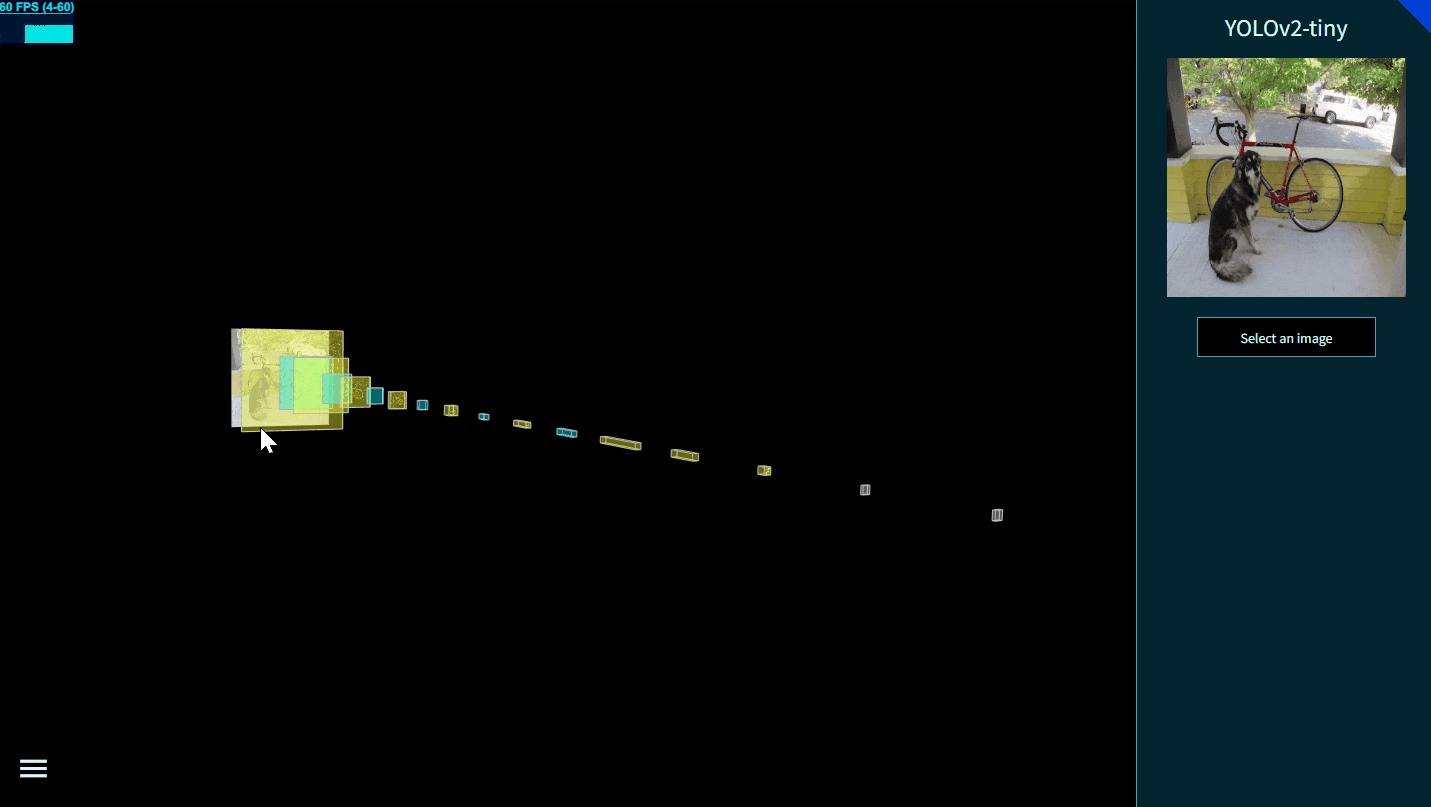Fig. 8 - Interactive Yolov2-tiny created by TensorSpace

• ResNet-50 [ Keras model ]

➡ Live Demo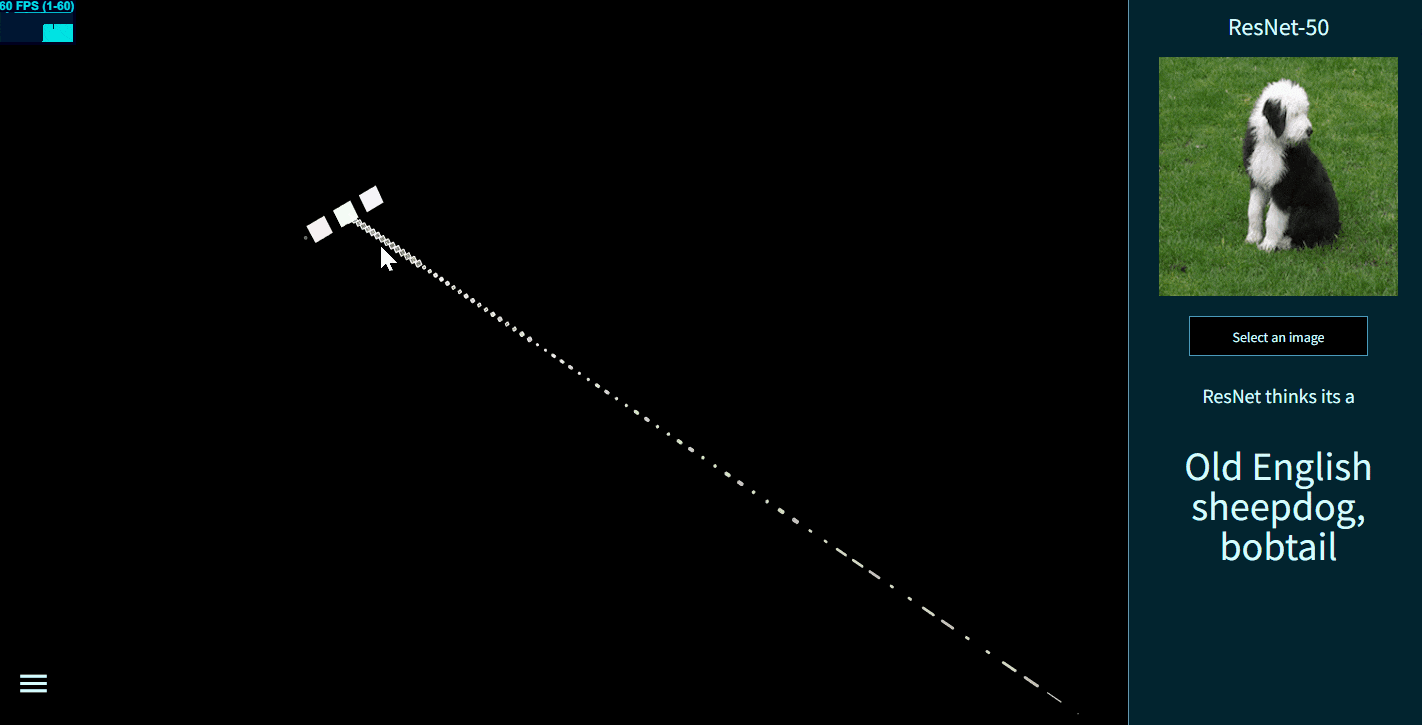Fig. 9 - Interactive ResNet-50 created by TensorSpace

• Vgg16 [ Keras model ]

➡ Live Demo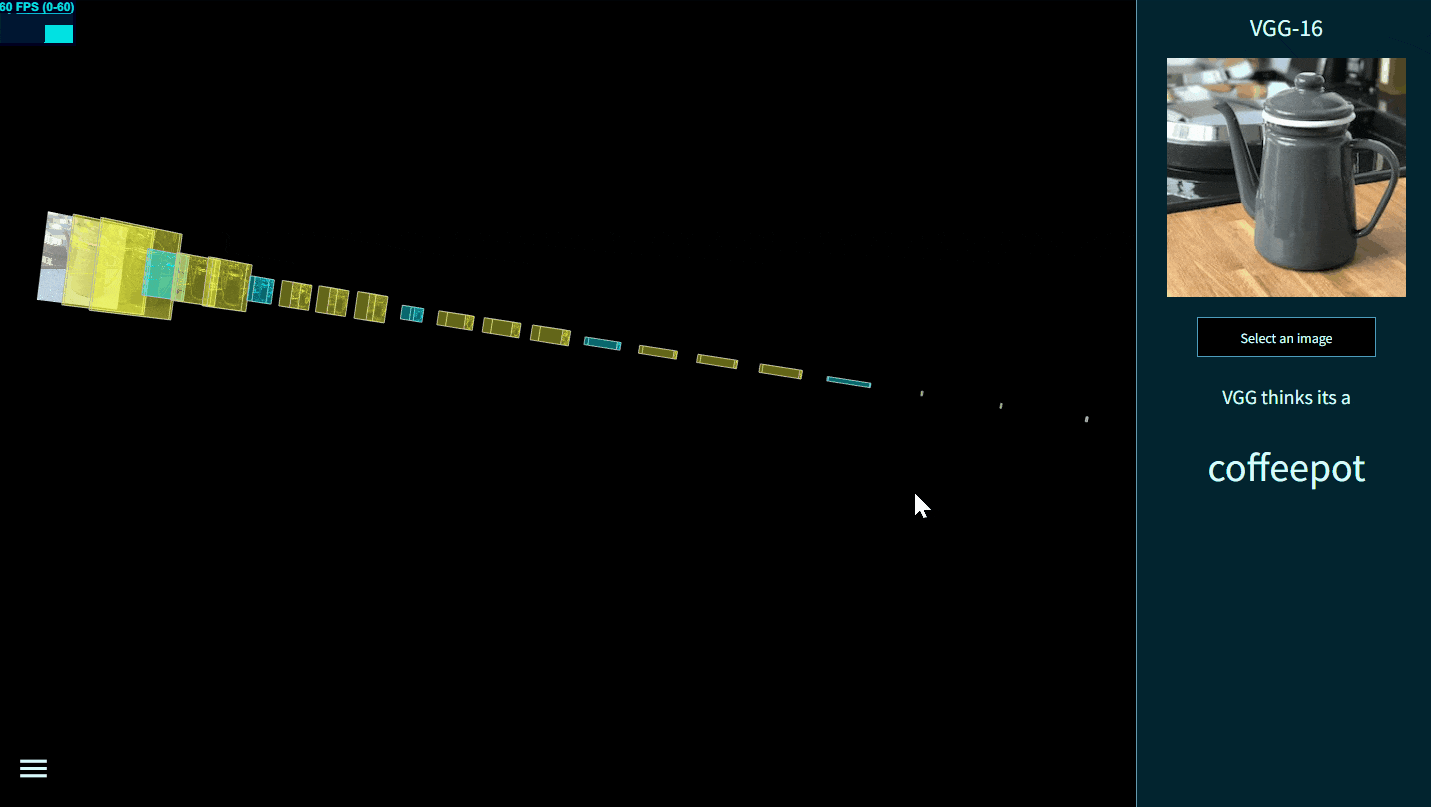Fig. 10 - Interactive Vgg16 created by TensorSpace

• ACGAN [ Keras model ]

➡ Live Demo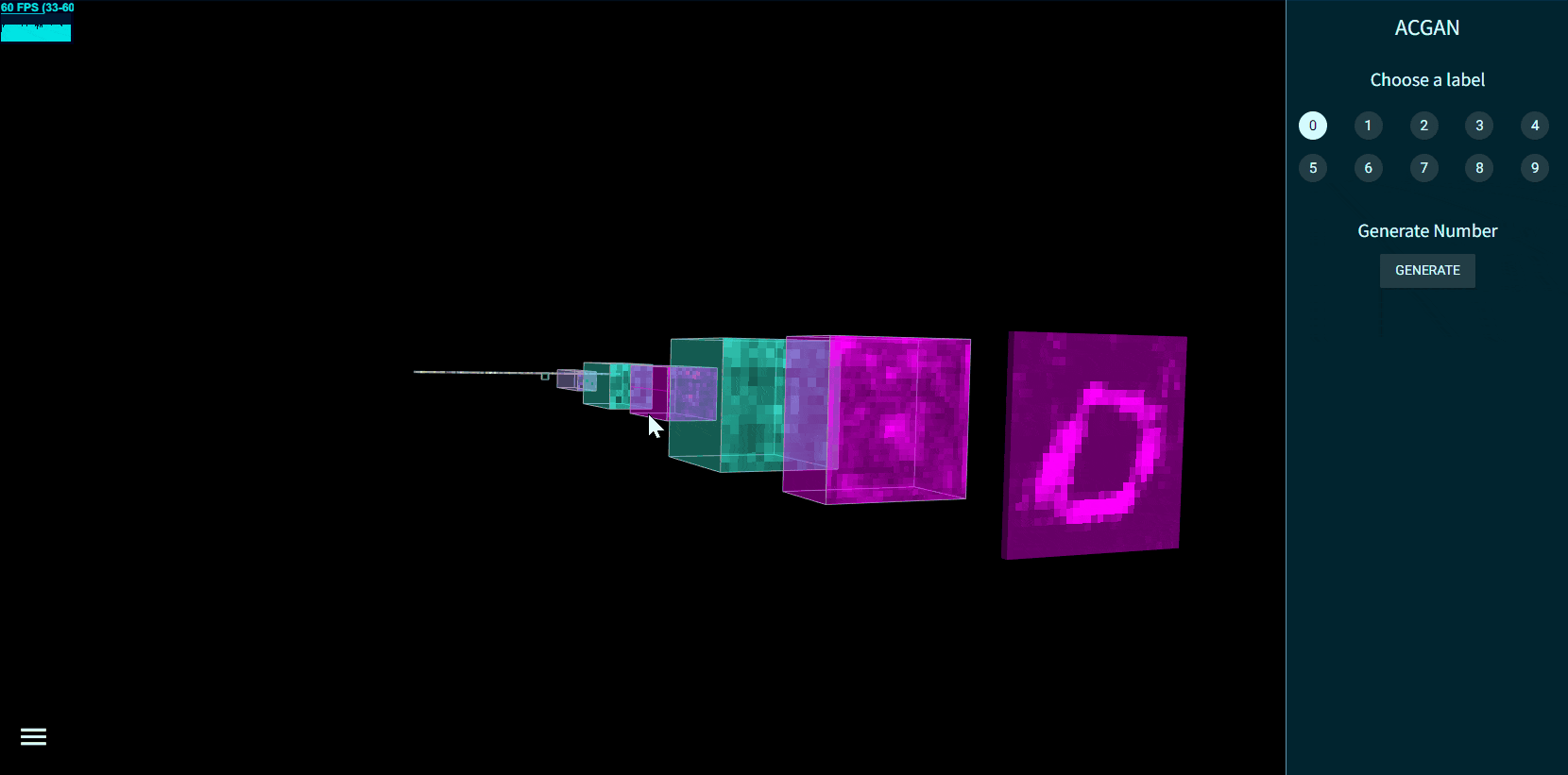Fig. 11 - Interactive ACGAN created by TensorSpace

• MobileNetv1 [ Keras model ]

➡ Live Demo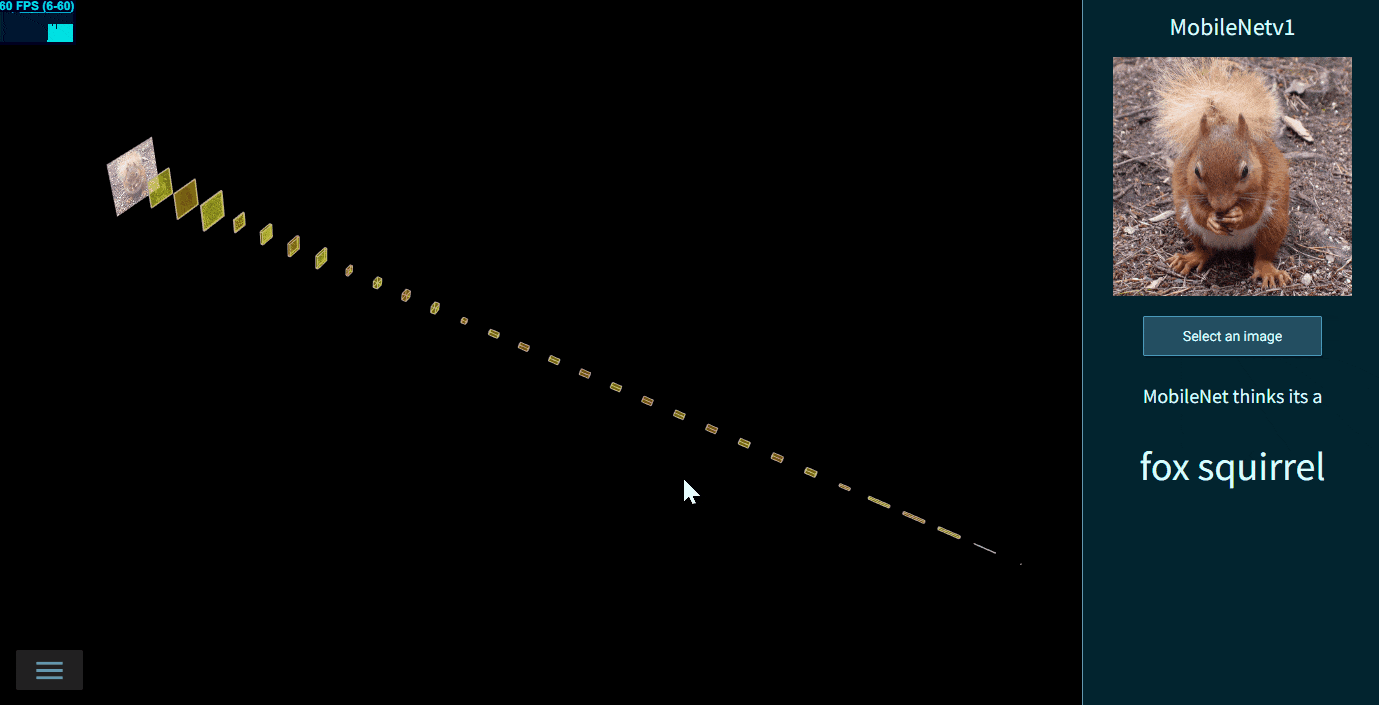Fig. 12 - Interactive MobileNetv1 created by TensorSpace

• Inceptionv3 [ Keras model ]

➡ Live Demo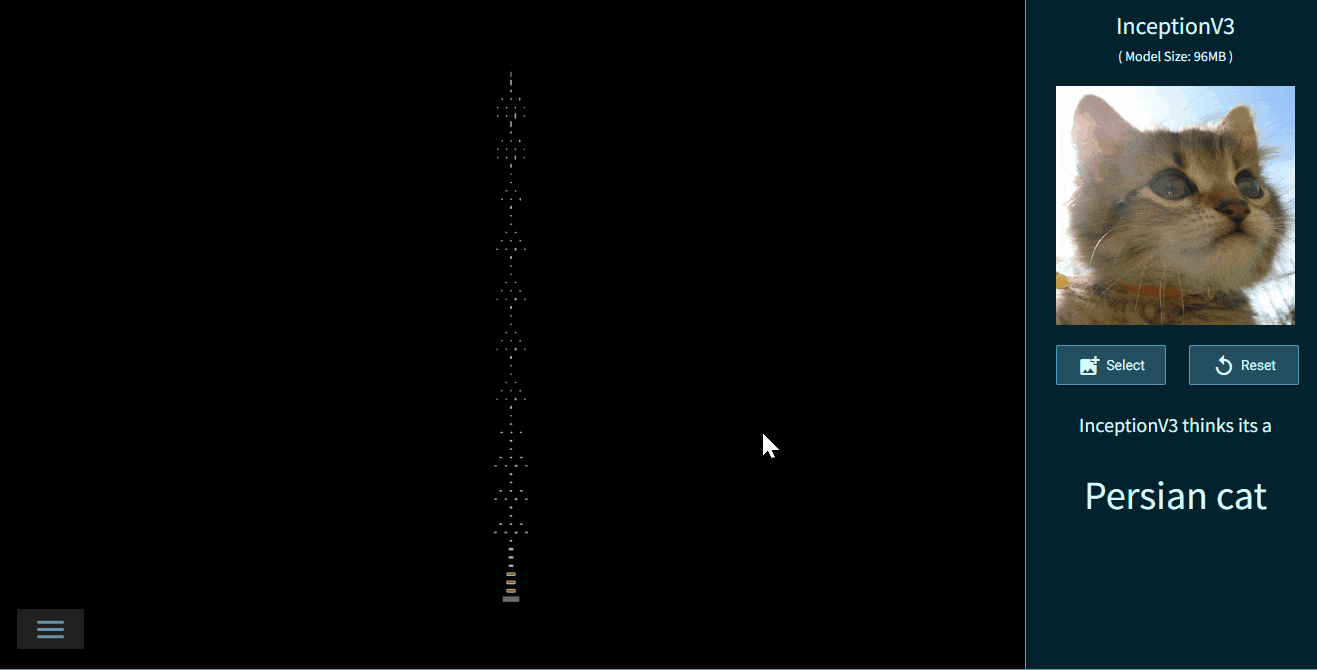Fig. 13 - Interactive Inceptionv3 created by TensorSpace

• LeNet Training Visualization [ TensorFlow.js dynamic model ]

Visualize the LeNet Training Process with TensorSpace.js and TensorFlow.js.

➡ Live Demo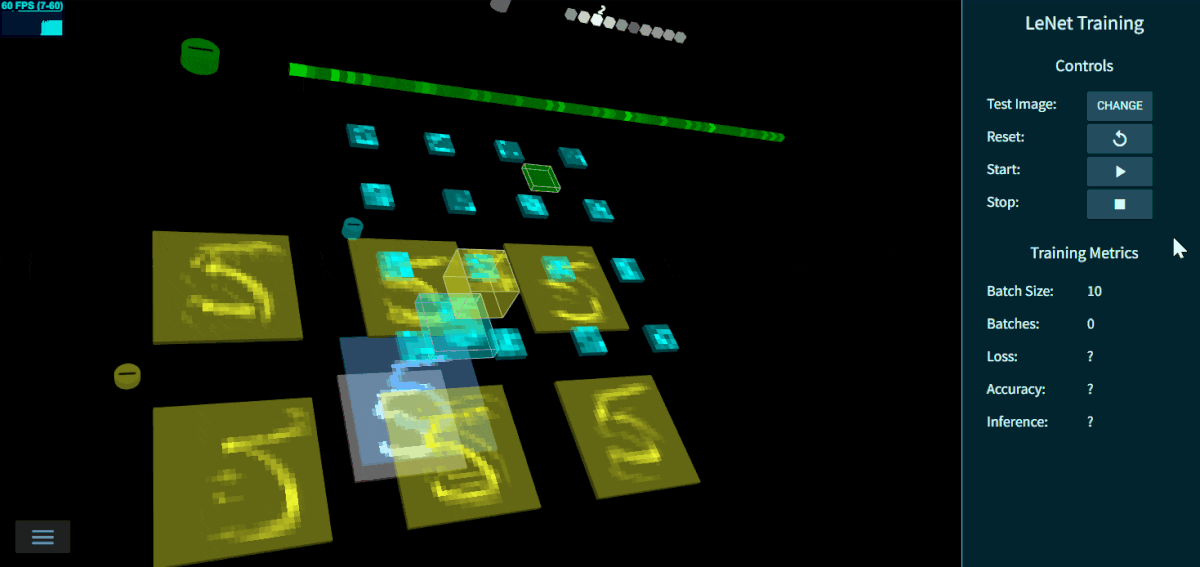Fig. 14 - LeNet Training 3D Visualization

### View models locally

As some models above are extremely large, view them locally may be a good choice.

• Step 1:
`clone`
TensorSpace Repo
```git clone https://github.com/tensorspace-team/tensorspace.git
```
• Step 2:

Open "html" file in examples folder in local web server.

## Contributors

Thanks goes to these wonderful people (emoji key):

## Contact

If you have any issue or doubt, feel free to contact us by: * Email: t[email protected] * GitHub Issues: create issue * Slack: #questions * Gitter: #Lobby

## Next Episode### TensorSpace-VR

Present Neural Network in VRFig. 15 - TensorSpace VR Demo